# Parallel Flows

Equations (2.116), (2.118), and (2.120) yield

 0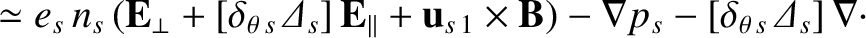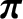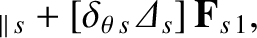(2.163) 0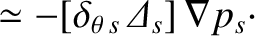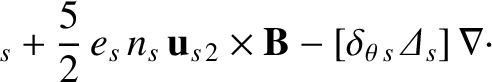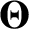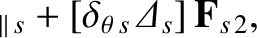(2.164)

where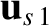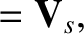(2.165)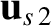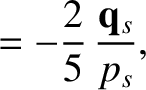(2.166)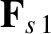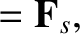(2.167)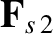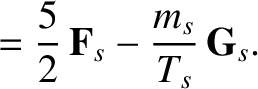(2.168)

Here, we have neglected terms that are second order in the small parameter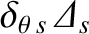. By analogy with the analysis Section 2.11, we can write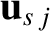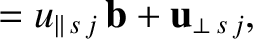(2.169)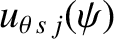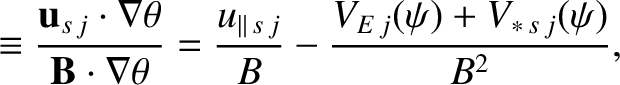(2.170)

for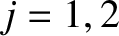, where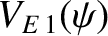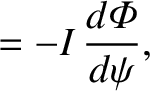(2.171)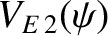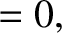(2.172)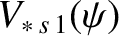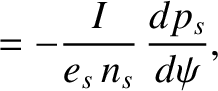(2.173)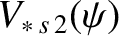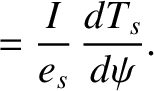(2.174)

Taking the scalar product of Equations (2.163) and (2.164) with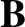, and flux-surface averaging, we annihilate the leading-order terms, to leave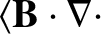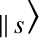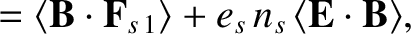(2.175)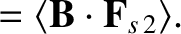(2.176)

Note that the annihilation of the term involving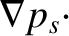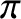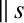in Equation (2.164) depends on the fact thattakes the Chew-Goldberger-Low form. [See Equation (2.154).]

According to Equations (2.175) and (2.176), the parallel components of the friction force density and the electric force density drive both particle flows and heat flows along the magnetic field. These flows are opposed by the parallel viscous force density. We now need to solve the previous two equations to determine the parallel flows, which requires us to have expressions for the friction force density, as well as the parallel viscous force density.